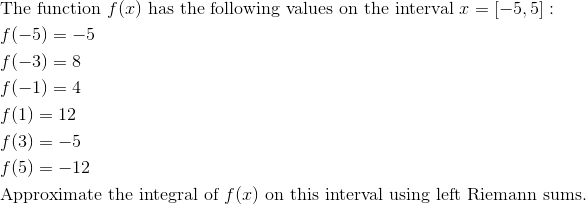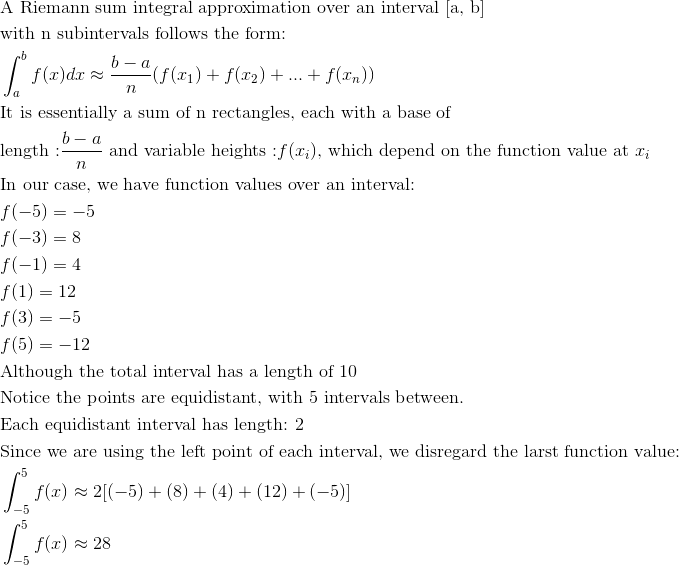AP Calculus AB : Riemann sums (left, right, and midpoint evaluation points)

Example Questions

Example Question #21 : Numerical Approximations To Definite Integrals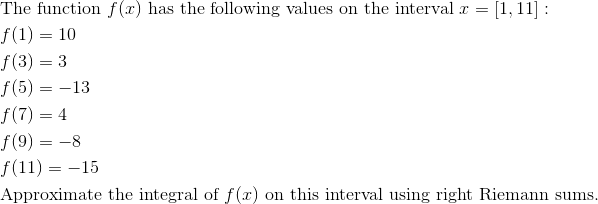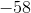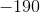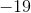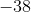Explanation: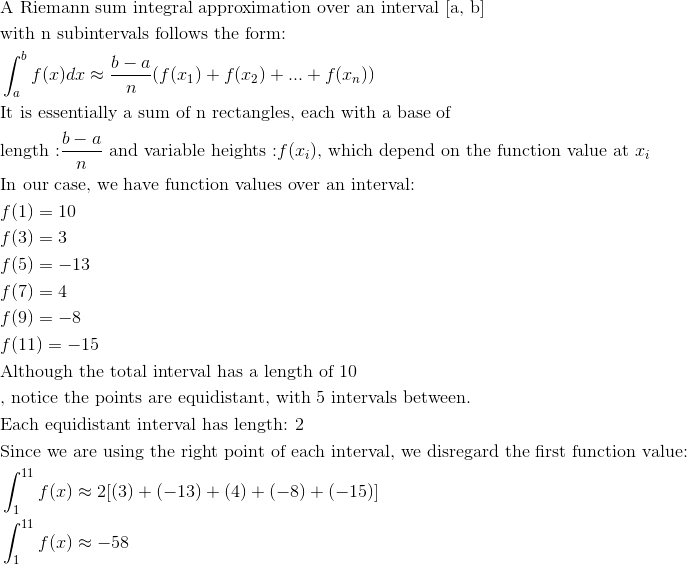Example Question #191 : Integrals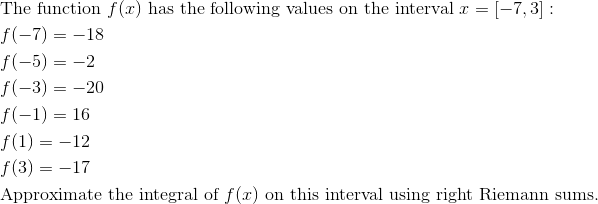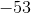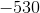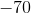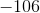Explanation: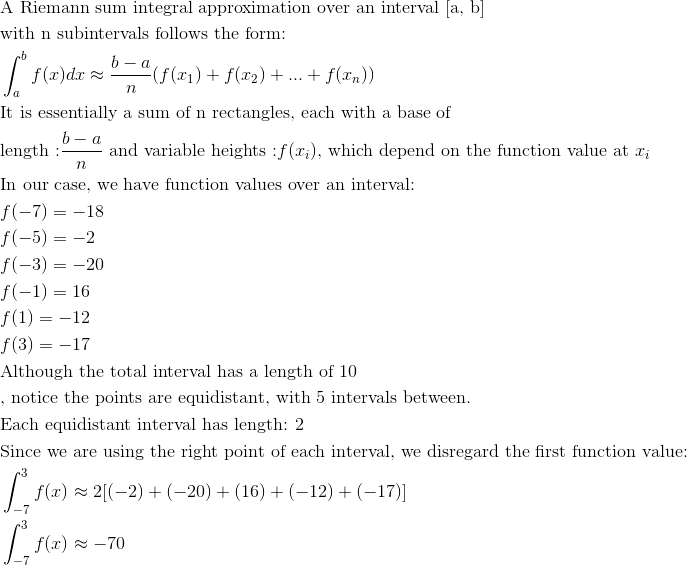Example Question #13 : Riemann Sums (Left, Right, And Midpoint Evaluation Points)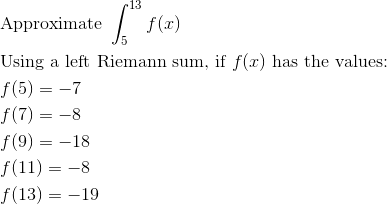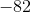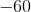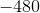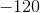Explanation: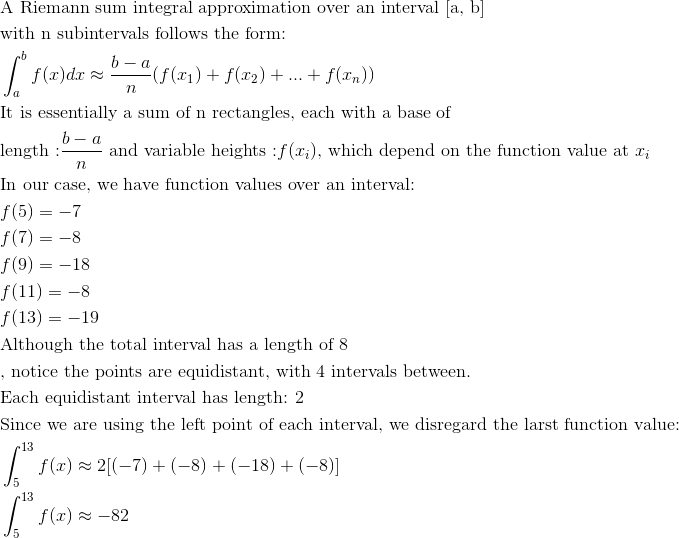Example Question #14 : Riemann Sums (Left, Right, And Midpoint Evaluation Points)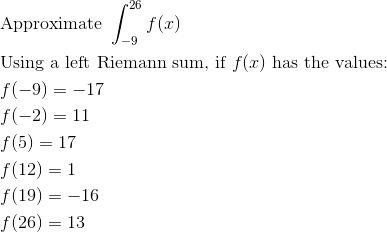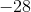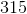Explanation: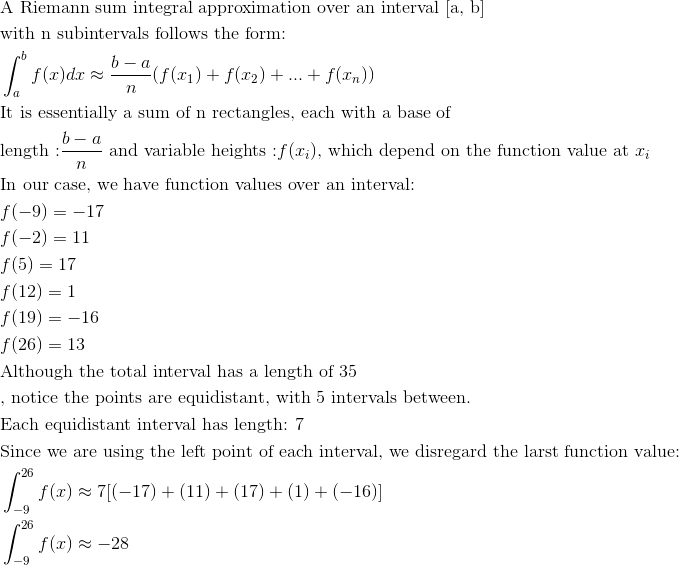Example Question #15 : Riemann Sums (Left, Right, And Midpoint Evaluation Points)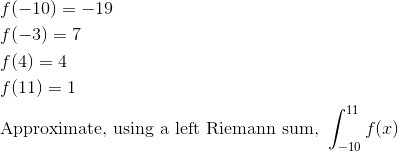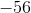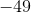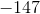Explanation: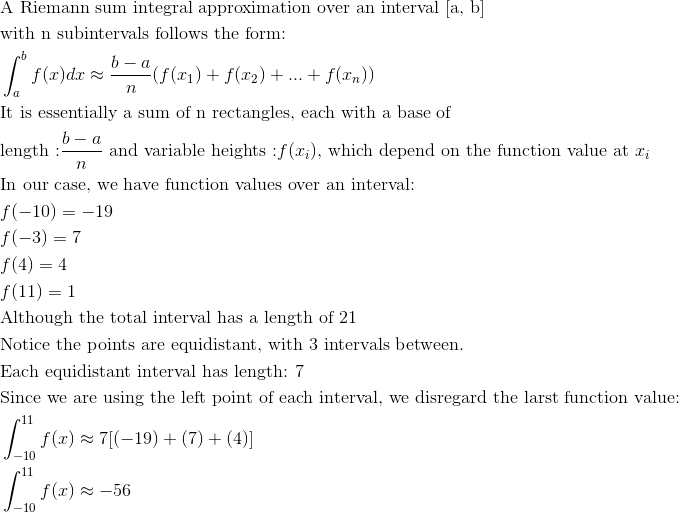Example Question #16 : Riemann Sums (Left, Right, And Midpoint Evaluation Points)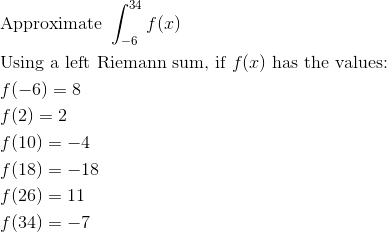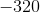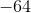Explanation: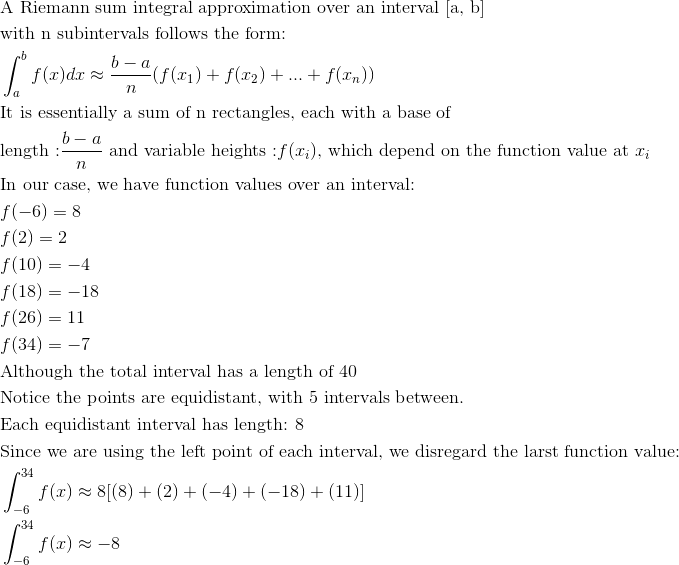Example Question #17 : Riemann Sums (Left, Right, And Midpoint Evaluation Points)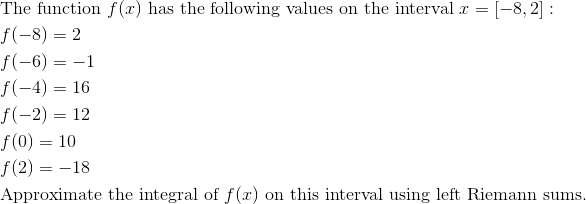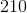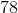Explanation: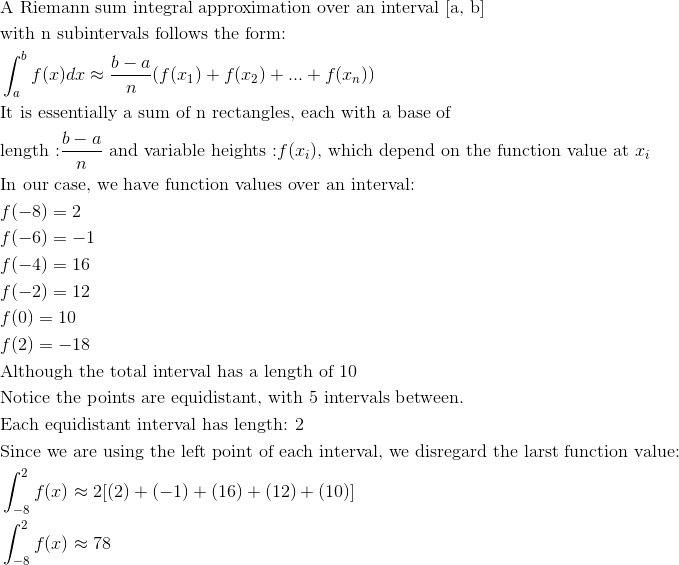Example Question #18 : Riemann Sums (Left, Right, And Midpoint Evaluation Points)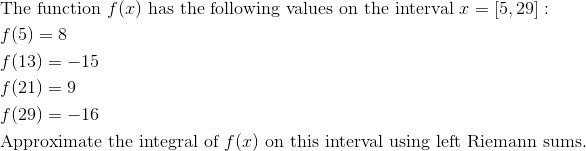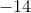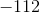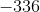Explanation: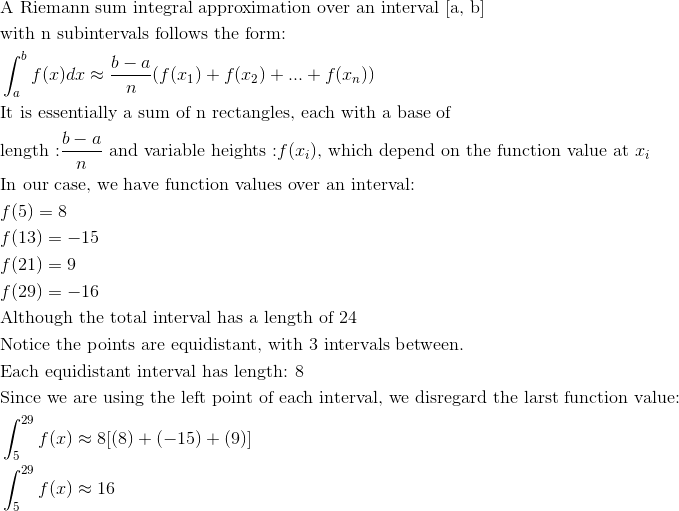Example Question #19 : Riemann Sums (Left, Right, And Midpoint Evaluation Points)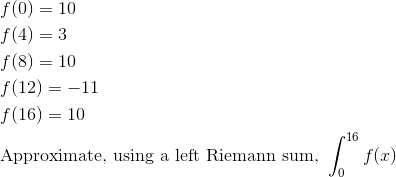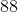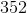Explanation: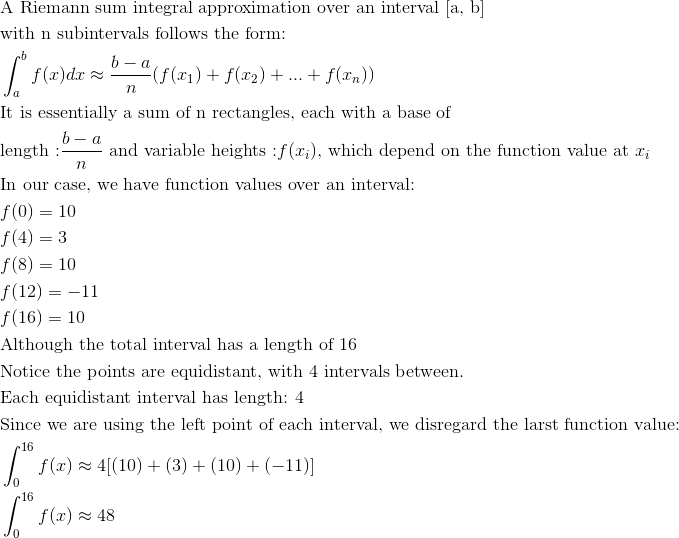Example Question #31 : Numerical Approximations To Definite Integrals